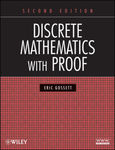## Faculty Books#### Title

Discrete mathematics with proof second edition

#### Description

Discrete mathematics has become increasingly popular in recent years due to its growing applications in the field of computer science. Discrete Mathematics with Proof, Second Edition continues to facilitate an up-to-date understanding of this important topic, exposing readers to a wide range of modern and technological applications. The book begins with an introductory chapter that provides an accessible explanation of discrete mathematics. Subsequent chapters explore additional related topics including counting, finite probability theory, recursion, formal models in computer science, graph theory, trees, the concepts of functions, and relations.

#### Department(s)

Mathematics and Computer Science

978047045793-1

2009

Wiley

Hoboken

#### Keywords

Discrete mathematics, Mathematical proofs

#### Disciplines

Discrete Mathematics and CombinatoricsCOinS